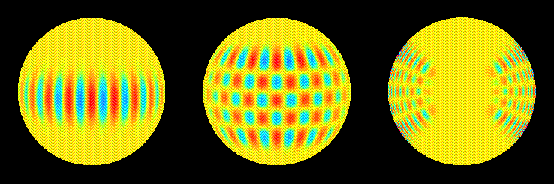# P-mode Shapes

The Sun is ringing, not like a bell struck by one clapper, but instead like a bell struck by many tiny sand grains. The Sun acts as a resonating cavity, exhibiting millions of oscillation modes or standing waves.

Most people are familiar with oscillating modes in one dimension: if you shake one end of a fairly tight rope you can set up an oscillating wave pattern that vibrates the whole rope back and forth together. If you shake faster, stable patterns with two, three, or more oscillations along the rope can be established. In these overtone patterns are places in the rope that don't move, those are called nodes. The patterns can be described mathematically with sine waves of only certain wavelengths depending on the length of the rope.

In two dimensions, more modes of vibration are possible. Try putting a coffee mug on the counter when the garbage disposal is running, and you may be able to see a fairly complex two dimensional pattern on the surface with nodes lying along certain fixed curves. Mathematical physicists typically use Bessel functions to describe these patterns.

In three dimensions modes can be described in terms of mathematical functions called spherical harmonics. Spherical harmonics have characteristic spatial patterns with nodes located both on the Sun's surface and radially within the Sun.

The three different kinds of waves that helioseismologists look for generate p, g, and f modes as resonant modes of oscillation. There are about 10^7 p and f modes alone. [Harvey, 1995, pp. 33].

The p modes can be described in terms of spherical harmonics. The spherical harmonics are characterized by three whole numbers: The order, given by n, is the number of nodes in the radial direction. The harmonic degree, called l, indicates the number of node lines on the surface, which is the total number of planes slicing through the Sun. The third number, m, tells how many of the surface nodal lines cross the equator and also gives the phase; m runs from -l to +l because the direction of the waves can be important. The number m, describes the number of planes slicing through the Sun longitudinally.

You can think of the numbers l and m describing a checkerboard pattern on the surface of a globe. The grid pattern is defined by 'm' circles passing through the pole, like longitude meridians, and by l-m lines corresponding to parallels of latitude. The order n tells how many times the pattern reverses itself inside the Sun between the surface and center.

In the left panel of the top figure: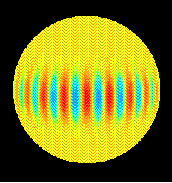we show the surface pattern of an l=19, m=19 mode. There are 19 circles passing through the north pole and crossing the equator. The p mode with this spatial pattern causes motion of the surface that alternate in sign (in or out of the surface) in adjacent regions. The difference between m=+/-18 is the phase (in or out) of the pattern.

In the middle panel of the top figure: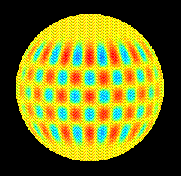we show and l=19, m=15. Here there are 15 meridional circles of nodes and 4 lines in the east-west direction. The checkerboard pattern shows where material would move in or out at a given time due to the mode.

The number of radial nodes n in these two cases was not specified. The number n determines how many nodes exist on a line from the center of the Sun to its surface, and does not influence the surface appearance for the mode.

In the right panel of the top figure: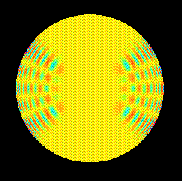we show a cutaway view of the Sun's interior showing how the n=11, l=19, m=15 mode would look with depth. There are 11 reversals of the surface pattern between the surface and the core.

Each mode is sensitive to the physical conditions where the amplitudes of the mode are greatest. It is easy to see that the various modes overlap, but that each one is sensitive to different parts of the solar interior. By combining a large number of modes one can build up a consistent picture of what is happening throughout the solar interior. It is interesting to note that none of these modes is sensitive at the Sun poles. That is because all of the node lines of zero amplitude converge at high latitude. The m=0 modes look like horizontal bands, and only those have any sensitivity at 90 degrees. There is a simlar problem with probing the deepest interior. Only low order, low degree modes have any chance of sensing what is happening in the core.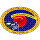SOI